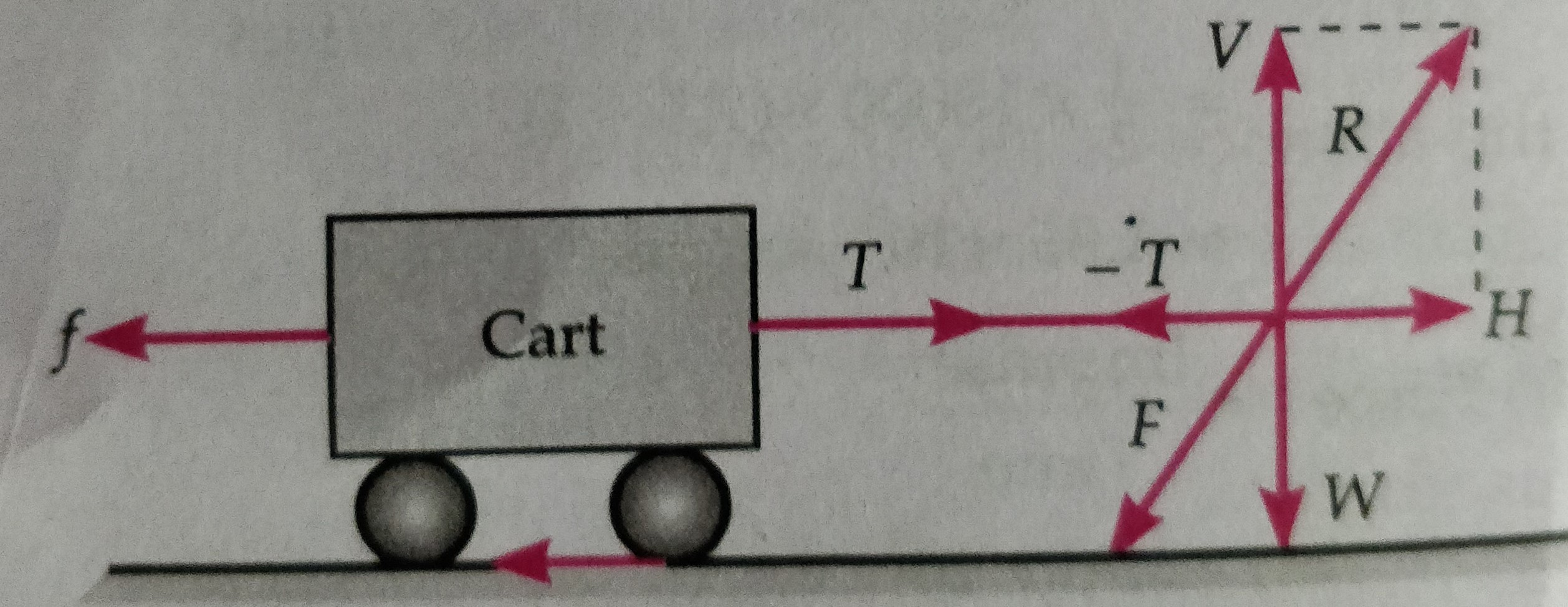# Horse and cart problem

This is the topic  Horse and cart problem which explain the one of the important illustration of Newton’s third law of motion.

But before to learn the topic Horse and cart problem students must know, Newton’s third law of motion. To get the notes on Newton’s third law of motion click here-

To get the Notes on Newton’s first law and inertia click here-

To get the Notes on Newton’s second law and impulse click here-

Horse and cart problems –

Suppose a cart is connected  with a  horse by a light inextensible string. When horse start to walk horse press the ground F ( action ) with their feet in the slightly backward direction and ground  exert an equal reaction R , as shown in figure . then R has two component H = R cosϴ in horizontal direction  and V= R sinϴ in vertical direction . V balance the weight of the horse and H makes the horse to move in forward direction . While pulling the cart produces a tension T in the string .If H > T then cart starts moving in the forward direction with acceleration ‘a’ .

Net force acting on the horse = H-T= ma.( where m is the mass of the horse)……………..(i)

If f is the frictional force between tyre of cart and ground then for cart we can write

T-F= Ma  ( M is the mass of the cart ) …………………………(ii)

From equations (i) and (ii) we get ,

H-f = ( M+m ) a ;

So, acceleration   a  =  H-f/ (M+m ) ;

The whole system will move if H>f ;

### 6 thoughts on “Horse and cart problem”

• July 30, 2020 at 3:59 am

Like!! Really appreciate you sharing this blog post.Really thank you! Keep writing.

• July 30, 2020 at 4:00 am

Thank you ever so for you article post.

• July 30, 2020 at 4:01 am

A big thank you for your article.

• July 30, 2020 at 4:05 am

I am regular visitor, how are you everybody? This article posted at this web site is in fact pleasant.